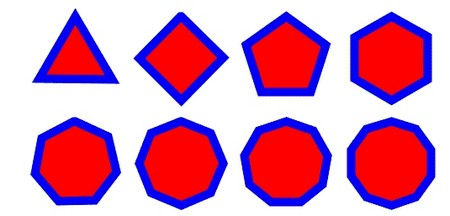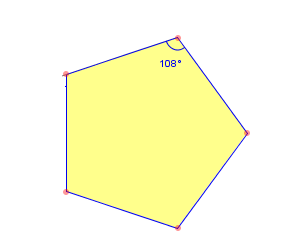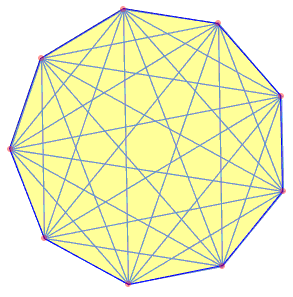## Types of Polygon

Published on: Mar 21 2011 by Shenron

In traditional geometry, polygon is a flat shape that is normally 2-dimensional. Polygons are made up of straight lines which are connected with each other or in simple words polygon is a flat shape that is enclosed by straight line segments. These segments are referred to as its edges or sides and where these segments come into contact with each other are known as its vertices or corners. The interior of the polygon is often referred to as its body.

The term polygon is derived from Greek ????? (polús) which means “much”, “many” and ????? (g?nía) which means “corner” or “angle”. Nowadays, polygon is often studied in terms of its sides.

To qualify as a polygon, it is necessary that the angle made by connecting two edges must not be 180°. Following are given some of the different types of polygon.Triangle

A triangle is a type of polygon having three corners (vertices) and three sides (edges) which are line segments and not the curved lines. Normally, a triangle is symbolized as  ABC (three vertices).

A quadrilateral is a type of polygon having four sides or edges and four corners or vertices. The term quadrilateral is a combination of two terms, i.e. ‘quad’ which means ‘four’ and ‘lateral’ which means ‘of sides’. Quadrilateral can be of two types: simple quadrilateral and complex quadrilateral. The former can be either concave or convex and are not self-intersecting, while the latter are self intersecting and are often referred to as crossed.

Pentagon

Pentagon is derived from a Greek word ‘pente’ which means five. Geometrically, a pentagon is a type of polygon having five sides or edges and five corners or vertices. It can either be not self- intersecting (simple) or self intersecting such as a pentagram. All the internal five angles have a sum equal to 540°.Hexagon

The term hexagon is taken from Greek ?? hex, ‘six’ which means six. A hexagon is a geometrical shape having six sides or edges and six corners or vertices. The sum of internal angles for hexagon is 720°. Its Schläfli symbol is {6}.

Heptagon

It is a geometrical shape having seven sides or edges and seven vertices. It has a Schläfli symbol {7}. In a regular heptagon, the sum of measure of all the angles is 128.5714286° and the sides or edges of the regular heptagon meet at an angle of 5?/7.

Octagon

An octagon is a type of polygon having eight sides and eight corners. It has a Schläfli symbol {8}. A regular octagon usually has all the eight sides of equal length and internal angles also equal. Each internal angle of octagon measures 135° and hence, the sum of measure of all the internal angles of octagon is 1080°.

Nonagon

It is a type of polygon having nine sides and nine corners. The term nonagon is derived from the Latin word ‘nonus’ which means ‘nine’ and ‘gonon’ means ‘sides’. This term was first coined in French in 16th century as nonogone and later in English in 17th century. Nonagon is also known as enneagon which is derived from Greek word enneagonon. ?????, means ‘nine’ plus ?????, from ?????, which means ‘corner’.Decagon

Decagon is a geometrical shape having ten sides or edges and ten corners or vertices. A regular decagon has all its ten sides of equal length with an internal angle measure as 144°. It has a Schläfli symbol {10}.

Dodecagon

Dodecagon is a kind of polygon having twelve sides or edges and twelve corners or vertices. A regular dodecagon is a geometrical shape with a sum of measure of all the sides and angles equal. An internal angle of a dodecagon is of 150°. It has a Schläfli symbol {12}.